# Transfer learning for video classification with MoViNet

MoViNets (Mobile Video Networks) provide a family of efficient video classification models, supporting inference on streaming video. In this tutorial, you will use a pre-trained MoViNet model to classify videos, specifically for an action recognition task, from the UCF101 dataset. A pre-trained model is a saved network that was previously trained on a larger dataset. You can find more details about MoViNets in the MoViNets: Mobile Video Networks for Efficient Video Recognition paper by Kondratyuk, D. et al. (2021). In this tutorial, you will:

• Create a new model using a pre-trained model with a new classifier by freezing the convolutional base of the MoViNet model
• Replace the classifier head with the number of labels of a new dataset
• Perform transfer learning on the UCF101 dataset

The model downloaded in this tutorial is from official/projects/movinet. This repository contains a collection of MoViNet models that TF Hub uses in the TensorFlow 2 SavedModel format.

This transfer learning tutorial is the third part in a series of TensorFlow video tutorials. Here are the other three tutorials:

• Load video data: This tutorial explains much of the code used in this document; in particular, how to preprocess and load data through the `FrameGenerator` class is explained in more detail.
• Build a 3D CNN model for video classification. Note that this tutorial uses a (2+1)D CNN that decomposes the spatial and temporal aspects of 3D data; if you are using volumetric data such as an MRI scan, consider using a 3D CNN instead of a (2+1)D CNN.
• MoViNet for streaming action recognition: Get familiar with the MoViNet models that are available on TF Hub.

## Setup

Begin by installing and importing some necessary libraries, including: remotezip to inspect the contents of a ZIP file, tqdm to use a progress bar, OpenCV to process video files (ensure that `opencv-python` and `opencv-python-headless` are the same version), and TensorFlow models (`tf-models-official`) to download the pre-trained MoViNet model. The TensorFlow models package are a collection of models that use TensorFlow’s high-level APIs.

````pip install remotezip tqdm opencv-python==4.5.2.52 opencv-python-headless==4.5.2.52 tf-models-official`
```
``````import tqdm
import random
import pathlib
import itertools
import collections

import cv2
import numpy as np
import remotezip as rz
import seaborn as sns
import matplotlib.pyplot as plt

import keras
import tensorflow as tf
import tensorflow_hub as hub
from tensorflow.keras import layers
from tensorflow.keras.losses import SparseCategoricalCrossentropy

# Import the MoViNet model from TensorFlow Models (tf-models-official) for the MoViNet model
from official.projects.movinet.modeling import movinet
from official.projects.movinet.modeling import movinet_model
``````

The hidden cell below defines helper functions to download a slice of data from the UCF-101 dataset, and load it into a `tf.data.Dataset`. The Loading video data tutorial provides a detailed walkthrough of this code.

The `FrameGenerator` class at the end of the hidden block is the most important utility here. It creates an iterable object that can feed data into the TensorFlow data pipeline. Specifically, this class contains a Python generator that loads the video frames along with its encoded label. The generator (`__call__`) function yields the frame array produced by `frames_from_video_file` and a one-hot encoded vector of the label associated with the set of frames.

``````URL = 'https://storage.googleapis.com/thumos14_files/UCF101_videos.zip'
num_classes = 10,
splits = {"train": 30, "test": 20},
``````
```train :
100%|██████████| 300/300 [00:31<00:00,  9.60it/s]
test :
100%|██████████| 200/200 [00:23<00:00,  8.69it/s]
```

Create the training and test datasets:

``````batch_size = 8
num_frames = 8

output_signature = (tf.TensorSpec(shape = (None, None, None, 3), dtype = tf.float32),
tf.TensorSpec(shape = (), dtype = tf.int16))

train_ds = tf.data.Dataset.from_generator(FrameGenerator(subset_paths['train'], num_frames, training = True),
output_signature = output_signature)
train_ds = train_ds.batch(batch_size)

test_ds = tf.data.Dataset.from_generator(FrameGenerator(subset_paths['test'], num_frames),
output_signature = output_signature)
test_ds = test_ds.batch(batch_size)
``````

The labels generated here represent the encoding of the classes. For instance, 'ApplyEyeMakeup' is mapped to the integer Take a look at the labels of the training data to ensure that the dataset has been sufficiently shuffled.

``````for frames, labels in train_ds.take(10):
print(labels)
``````
```tf.Tensor([0 9 9 4 8 6 1 6], shape=(8,), dtype=int16)
tf.Tensor([0 4 3 2 9 1 6 2], shape=(8,), dtype=int16)
tf.Tensor([1 5 7 6 3 4 9 6], shape=(8,), dtype=int16)
tf.Tensor([1 6 9 1 3 9 9 0], shape=(8,), dtype=int16)
tf.Tensor([3 2 2 8 5 1 5 1], shape=(8,), dtype=int16)
tf.Tensor([5 2 3 3 7 3 3 0], shape=(8,), dtype=int16)
tf.Tensor([2 4 1 6 7 6 6 1], shape=(8,), dtype=int16)
tf.Tensor([7 4 5 2 9 2 2 5], shape=(8,), dtype=int16)
tf.Tensor([1 7 0 8 5 7 6 7], shape=(8,), dtype=int16)
tf.Tensor([7 7 8 3 0 6 5 0], shape=(8,), dtype=int16)
```

Take a look at the shape of the data.

``````print(f"Shape: {frames.shape}")
print(f"Label: {labels.shape}")
``````
```Shape: (8, 8, 224, 224, 3)
Label: (8,)
```

## What are MoViNets?

As mentioned previously, MoViNets are video classification models used for streaming video or online inference in tasks, such as action recognition. Consider using MoViNets to classify your video data for action recognition.

A 2D frame based classifier is efficient and simple to run over whole videos, or streaming one frame at a time. Because they can't take temporal context into account they have limited accuracy and may give inconsistent outputs from frame to frame.

A simple 3D CNN uses bidirectional temporal context which can increase accuracy and temporal consistency. These networks may require more resources and because they look into the future they can't be used for streaming data.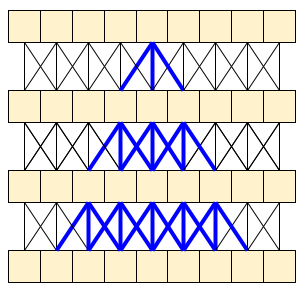The MoViNet architecture uses 3D convolutions that are "causal" along the time axis (like `layers.Conv1D` with `padding="causal"`). This gives some of the advantages of both approaches, mainly it allow for efficient streaming.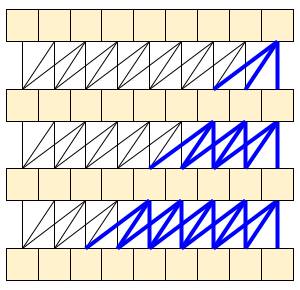Causal convolution ensures that the output at time t is computed using only inputs up to time t. To demonstrate how this can make streaming more efficient, start with a simpler example you may be familiar with: an RNN. The RNN passes state forward through time: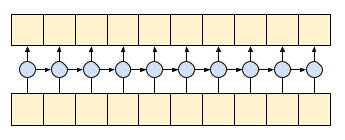``````gru = layers.GRU(units=4, return_sequences=True, return_state=True)

inputs = tf.random.normal(shape=[1, 10, 8]) # (batch, sequence, channels)

result, state = gru(inputs) # Run it all at once
``````

By setting the RNN's `return_sequences=True` argument you ask it to return the state at the end of the computation. This allows you to pause and then continue where you left off, to get exactly the same result: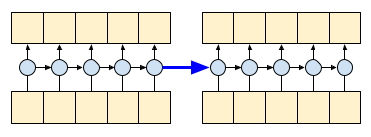``````first_half, state = gru(inputs[:, :5, :])   # run the first half, and capture the state
second_half, _ = gru(inputs[:,5:, :], initial_state=state)  # Use the state to continue where you left off.

print(np.allclose(result[:, :5,:], first_half))
print(np.allclose(result[:, 5:,:], second_half))
``````
```True
True
```

Causal convolutions can be used the same way, if handled with care. This technique was used in the Fast Wavenet Generation Algorithm by Le Paine et al. In the MoVinet paper, the `state` is referred to as the "Stream Buffer".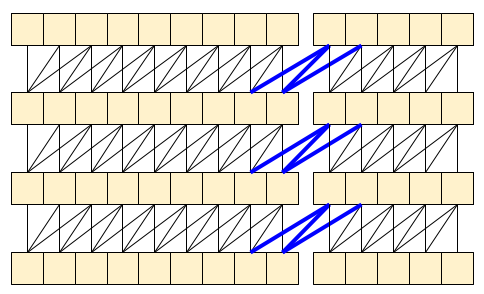By passing this little bit of state forward, you can avoid recalculating the whole receptive field that shown above.

In this section, you will:

1. You can create a MoViNet model using the open source code provided in `official/projects/movinet` from TensorFlow models.
3. Freeze the convolutional base, or all other layers except the final classifier head, to speed up fine-tuning.

To build the model, you can start with the `a0` configuration because it is the fastest to train when benchmarked against other models. Check out the available MoViNet models on TensorFlow Model Garden to find what might work for your use case.

``````model_id = 'a0'
resolution = 224

tf.keras.backend.clear_session()

backbone = movinet.Movinet(model_id=model_id)
backbone.trainable = False

# Set num_classes=600 to load the pre-trained weights from the original model
model = movinet_model.MovinetClassifier(backbone=backbone, num_classes=600)
model.build([None, None, None, None, 3])

!tar -xvf movinet_a0_base.tar.gz

checkpoint_dir = f'movinet_{model_id}_base'
checkpoint_path = tf.train.latest_checkpoint(checkpoint_dir)
checkpoint = tf.train.Checkpoint(model=model)
status = checkpoint.restore(checkpoint_path)
status.assert_existing_objects_matched()
``````
```WARNING:tensorflow:`tf.keras.layers.experimental.SyncBatchNormalization` endpoint is deprecated and will be removed in a future release. Please use `tf.keras.layers.BatchNormalization` with parameter `synchronized` set to True.
movinet_a0_base/
movinet_a0_base/checkpoint
movinet_a0_base/ckpt-1.data-00000-of-00001
movinet_a0_base/ckpt-1.index
```

To build a classifier, create a function that takes the backbone and the number of classes in a dataset. The `build_classifier` function will take the backbone and the number of classes in a dataset to build the classifier. In this case, the new classifier will take a `num_classes` outputs (10 classes for this subset of UCF101).

``````def build_classifier(batch_size, num_frames, resolution, backbone, num_classes):
"""Builds a classifier on top of a backbone model."""
model = movinet_model.MovinetClassifier(
backbone=backbone,
num_classes=num_classes)
model.build([batch_size, num_frames, resolution, resolution, 3])

return model
``````
``````model = build_classifier(batch_size, num_frames, resolution, backbone, 10)
``````

For this tutorial, choose the `tf.keras.optimizers.Adam` optimizer and the `tf.keras.losses.SparseCategoricalCrossentropy` loss function. Use the metrics argument to the view the accuracy of the model performance at every step.

``````num_epochs = 2

loss_obj = tf.keras.losses.SparseCategoricalCrossentropy(from_logits=True)

model.compile(loss=loss_obj, optimizer=optimizer, metrics=['accuracy'])
``````

Train the model. After two epochs, observe a low loss with high accuracy for both the training and test sets.

``````results = model.fit(train_ds,
validation_data=test_ds,
epochs=num_epochs,
validation_freq=1,
verbose=1)
``````
```Epoch 1/2
38/38 [==============================] - 88s 2s/step - loss: 0.8982 - accuracy: 0.8000 - val_loss: 0.1608 - val_accuracy: 0.9800
Epoch 2/2
38/38 [==============================] - 54s 1s/step - loss: 0.1073 - accuracy: 0.9833 - val_loss: 0.0893 - val_accuracy: 0.9750
```

## Evaluate the model

The model achieved high accuracy on the training dataset. Next, use Keras `Model.evaluate` to evaluate it on the test set.

``````model.evaluate(test_ds, return_dict=True)
``````
```25/25 [==============================] - 21s 840ms/step - loss: 0.0920 - accuracy: 0.9750
{'loss': 0.09201380610466003, 'accuracy': 0.9750000238418579}
```

To visualize model performance further, use a confusion matrix. The confusion matrix allows you to assess the performance of the classification model beyond accuracy. To build the confusion matrix for this multi-class classification problem, get the actual values in the test set and the predicted values.

``````def get_actual_predicted_labels(dataset):
"""
Create a list of actual ground truth values and the predictions from the model.

Args:
dataset: An iterable data structure, such as a TensorFlow Dataset, with features and labels.

Return:
Ground truth and predicted values for a particular dataset.
"""
actual = [labels for _, labels in dataset.unbatch()]
predicted = model.predict(dataset)

actual = tf.stack(actual, axis=0)
predicted = tf.concat(predicted, axis=0)
predicted = tf.argmax(predicted, axis=1)

return actual, predicted
``````
``````def plot_confusion_matrix(actual, predicted, labels, ds_type):
cm = tf.math.confusion_matrix(actual, predicted)
ax = sns.heatmap(cm, annot=True, fmt='g')
sns.set(rc={'figure.figsize':(12, 12)})
sns.set(font_scale=1.4)
ax.set_title('Confusion matrix of action recognition for ' + ds_type)
ax.set_xlabel('Predicted Action')
ax.set_ylabel('Actual Action')
plt.xticks(rotation=90)
plt.yticks(rotation=0)
ax.xaxis.set_ticklabels(labels)
ax.yaxis.set_ticklabels(labels)
``````
``````fg = FrameGenerator(subset_paths['train'], num_frames, training = True)
label_names = list(fg.class_ids_for_name.keys())
``````
``````actual, predicted = get_actual_predicted_labels(test_ds)
plot_confusion_matrix(actual, predicted, label_names, 'test')
``````
```25/25 [==============================] - 27s 809ms/step
```

## Next steps

Now that you have some familiarity with the MoViNet model and how to leverage various TensorFlow APIs (for example, for transfer learning), try using the code in this tutorial with your own dataset. The data does not have to be limited to video data. Volumetric data, such as MRI scans, can also be used with 3D CNNs. The NUSDAT and IMH datasets mentioned in Brain MRI-based 3D Convolutional Neural Networks for Classification of Schizophrenia and Controls could be two such sources for MRI data.

In particular, using the `FrameGenerator` class used in this tutorial and the other video data and classification tutorials will help you load data into your models.